# Solutions to SQA examination

## 2001

```Section A
1. A		11. C
2. A		12. E
3. E		13. D
4. C		14. E
5. D		15. D
6. C		16. D
7. B		17. C
8. B		18. B
9. D		19. B
10.A		20. E

Section B
21.a.i.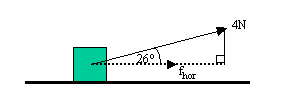Fhor= FResultantcosq
Fhor= 4cos26o
Fhor= 3.6N

a.ii. Fhor = Fun = 3.6N			a = Fun/m
m = 18kg			a = 3.6/18
a = ?				a = 0.2m/s2

a.iii.u = 0m/s			s = ut + 1/2at2
a = 0.2m/s2			s = 0x7 + 0.5x0.2x72
t = 7s				s = 4.9m
s = ?

b.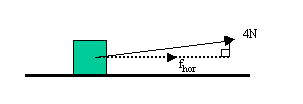By decreasing the angle the cosine of the angle will increase.
This makes the horizontal force greater as :Fhor= FResultantcosq
The accelerating force, and the consequent acceleration, is therefore greater.
Substituting a greater acceleration into the equation used in part a.iii. will
result in a greater distance being calculated.

22.a.i.							```
 Experiment Number 1 2 3 4 5 6 Mass of flask and air/kg 0.875 0.8762 0.8748 0.8755 0.876 0.8757 Mass of evacuated mask/kg 0.8722 0.8736 0.8721 0.8728 0.8738 0.8732 Mass of air removed/kg 0.0028 0.0026 0.0027 0.0027 0.0022 0.0025
```  a.ii.	Mean = Total/Number of readings
Mean = (0.0028+0.0026+0.0027+0.0027+0.0022+0.0025)/6
Mean = (0.0155/6)
Mean = 0.00258kg

Random uncertainty = (max - min)/N
Random uncertainty = (0.0028 - 0.0022)/6
Random uncertainty = 0.0001kg

Mass of air = (0.0026+-0.0001)kg

a.iii.	mass(mean) = 0.0026kg
volume = 2.0x10-3m3

density = mass/volume
r = m/V
r = 0.00258/2.0x10-3
r = 1.29kg/m3

a.iv.	A flask of larger volume is better because this increases the
mass and volume of air and used in the experiment. This should
result in a smaller percentage error in the measurements of
both mass and volume of the gas. This will in turn reduce the
percentage error in the calculated density of air.

22.bi.	Pressure and volume are the important variables.
This means Boyle's law requires to be used. However, as
it is the length of the cylinder that is given and not
the volume the fact that:
Volume(V) = Cylinder cross sectional area(A) x Length of the piston(L)
must be used in the solution.

V1 = L1A			V1/V2 = P1/P2
V2 = L2A			V1/V2 = L1A/L2A = L1/L2
P1 = 1x10-5Pa          =>L1/L2 = P1/P2
P2 = ?			P2 = (L2/L1)P1
P2 = (360/160)x1x10-5
P2 = 2.25x10-5Pa

b.ii.	The mass of the gas trapped is constant.

b.iii.Pressure is caused by the gas particles exerting a force on the
walls of the container. When the volume of the container decreases
there is an increase in the collision rate, meaning that more force
is exerted on the container walls. This increases the pressure as
pressure is a measure of force per unit area (P = F/A).

23.a.i.
(A)	Contact time(tc) = 3.0x10-3s
Favg = Fun = 0.5N

NB/ Favg = Fun because there are no unbalanced forces.

Impulse = Favg x tc
Impulse = 0.5 x 3.0x10-3
Impulse = 1.5x10-3Ns (kgm/s)

(B)	Impulse = Change in Momentum
Impulse = mv-mu
Impulse = m(v-u)

u = 0m/s  	As the bead of water is initially at rest.
m = 2.5x10-5kg

=> 	v = Impulse/m
v = 1.5x10-3/2.5x10-5
v = 60m/s

a.ii.	The impulse can be calculated from the area under the force
time graph.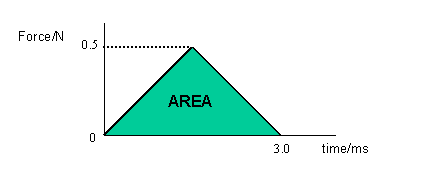A = 1/2bh (Area of a triangle formula)
A = 0.5 x 3.0x10-3 x 0.5
A = 0.75

=>	Actual impulse = 0.75x10-3Ns

v = Impulse/m  (from above)
v = 0.75x10-3/2.5x10-5
v = 30m/s

b.	The bead gains kinetic energy as it is accelerated in the
electric field between the plates. The gain in the kinetic
energy of the bead, with charge q, accelerated by a
potential difference V, can be calculated using:

Ek(gain) = qV

This is equal to the gain in kinetic energy:

Ek(gain) = mv2/2

=>mv2/2 = qV		q = 6.5x10-6C
v2 = 2qV/m		V = 5x103V
v = SQRT(2qV/m)		m = 4.0x10-5kg

v = SQRT(2x6.5x10-6x5x103)/4.0x10-5
v = SQRT(1625)
v = 40.3m/s

24.a.i. The emf can be found by projecting the graph line back until it
cuts the voltage axis.

emf = 4.8V

a.ii.	The internal resistance is equal to the negative of the gradient
of the line given.

m = (y1-y2)/(x1-x2)
m = (4.0-2.0)/(0.4-1.4)
m = 2.0/-1.0
m = -2

r = 2W

To justify the above consider:

y = mx + c  ...1
V = mI + c  ...2
Vtpd= E - Ir   ...3
Vtpd= -Ir + E  ...4

From equation (3)
When I = 0A :Vtpd=emf

Comparing (2) and (4)
m = -r

24.b.i.	emf = Vtpd+Vlost
emf = IR+Ir

The condition for a short circuit is: R=0W
=>	emf = Ir
I = emf/r
I = 12/0.050
I = 240A

b.ii.	When the lamp is connected: R=2.5W

I = emf/(R+r)
I = 12/(2.5+0.05)
I = 4.71A

P = I2R
P = (4.71)22.5
P = 55.46W

25.a.i.	The initial charging current(Imax) occurs when all of the supply
voltage(Vsupply) is across the 1.5kW resistor(R).

Imax = Vsupply/R
Imax = 6/1500
Imax = 4x10-3A

a.ii.	When fully charged the voltage across the supply voltage is equal to
the voltage across the capacitor.
Vsupply = Vc = 6V

Ecapacitor = QVc/2
Q = CVc
=>Ecapacitor = CVc2/2
Ecapacitor = 470x10-6x62/2
Ecapacitor = 8.46x10-3J

a.iii.	Increasing the supply voltage would increase the energy
storing capacity of the capacitor. This is because the
final voltage, across the fully charged capacitor, would
be higher.

25.b.	Etotal = 6.35x10-3J

fphoton = 5.80x1014Hz
h = 6.63x10-34Js
Ephoton = ?

Ephoton = hfphoton
Ephoton = 6.63x10-34 x 5.80x1014
Ephoton = 3.84x10-19J

Etotal = NEphoton
N = Etotal/Ephoton
N = 6.35x10-3/3.84x10-19
N = 1.65x1016

26.a.i.	The amplifier is acting in inverting mode.

a.ii.	The gain of the amplifier is calculated using the equation:

Voutput/Vinput = -Rfeedback/Rinput
Voutput = -VinputxRfeedback/Rinput
Voutput = -18-3x1.6x106/20x103

Voutput = -1.44V

a.iii.	The output voltage is not affected. This is because the output
voltage is still much lower than the supply voltage. Only when
the calculated output voltage gets close to, or greater than,
the supply voltage is the output voltage affected.

b.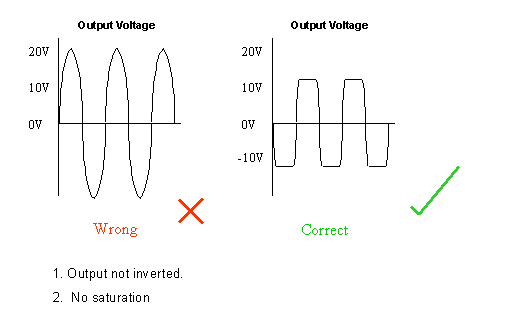27.a.	dsinq = nl

d = 2.16x10-6m
n = 2
l = 486x10-9m
q = ?

sinq = nl/d
sinq = 2x486x10-9/2.16x10-6
sinq = 0.45
q = 26.74o

b.i.	Angle i = 47o
Angle r = 27o

nglass = sin(i)/sin(r)
nglass = sin47o/sin27o
nglass = 0.731/0.454
nglass = 1.61	...as required

b.ii.	qcritical = sin-1(1/n)
qcritical = sin-1(1/1.61)
qcritical = sin-1(0.613)
qcritical = 38.4o

At point X the incident angle of 63o is greater than
the critical angle. This means that the light is totally
internally reflected at this boundary.

28.a.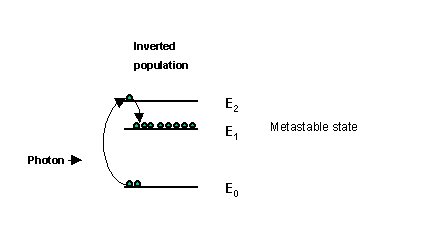A high voltage or other energy source can be  used to pump
electrons up into higher energy states. For example, an
electron can be pumped up to energy level (E2) and then
fall into the metastable state E1, creating what is called
an inverted population. A passing photon, having an energy equal
to the energy gap E1 to E0 can encourage/stimulate an electron
to drop from energy state E1 to E0 with  the production of a
photon in phase, with the same frequency and travelling
parallel to the stimulating photon. Thereafter, photons
produced by stimulated  emission can cause further stimulated
emission. This is the basis for stimulated emission and
amplification.

b.i.	P = IA

I = 1020Wm-2

A = pr2
A = px(5.00x10-4)2
A = 7.85x10-7m-2

P = 1020x7.854x10-7
P = 8.01x10-4W

b.ii.	The laser beam is non divergent. It does not spread out.
This means the radius of the spot is a constant.

29.a.i.	The reaction is induced fission. The reaction is
described as this type because the Pu nuclei absorb
neutrons and become unstable. The Pu nuclei then split
and release energy.

a.ii.	The energy released from the reaction is a result of
the mass of the products being less than the mass of
the reactants.

Mass of reactants = 3.9842x10-27kg
Mass of products = 3.9825x10-27kg

Mass defect = (3.9842-3.9825)x10-27kg
Mass defect = 0.0017x10-27kg

This mass defect is converted into energy.
The energy is calculated using the equation: E = mc2

E = 0.0017x10-27x(3x10-27)2
E = 1.53x10-13J

b.i.	D = E/m

E = 9.6x10-5J
m = 0.5kg

D = 9.6x10-5/0.5
D = 1.92x10-4J/kg (Gy)

b.ii.	H = QD

Q = 1
D = 1.92x10-4J/kg

H = 1x1.92x10-4
H = 1.92x10-4Sv

b.iii.	Three half value thicknesses of lead (120mm)
are required.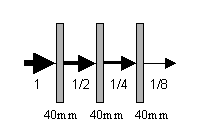END OF QUESTION PAPER